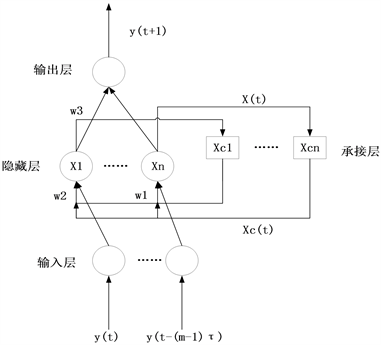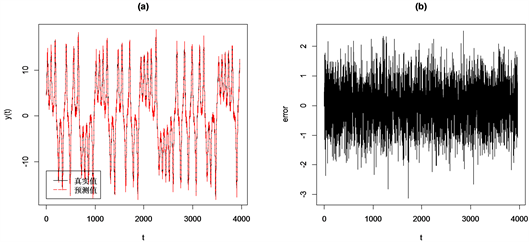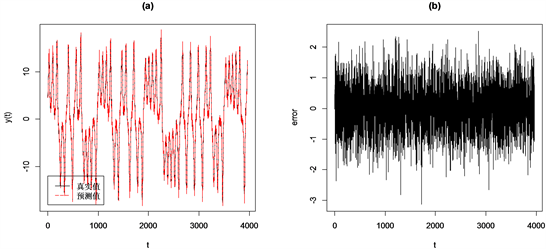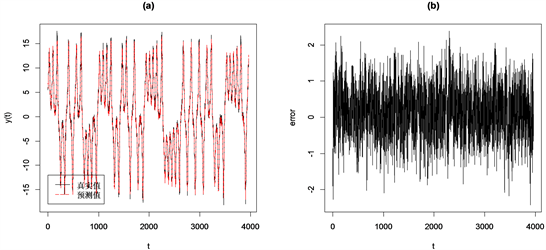# 基于混沌线性回归与Elman神经网络的组合预测Combined Prediction Based on Chaotic Linear Regression and Elman Neural Network

• 全文下载: PDF(1165KB)    PP.68-75   DOI: 10.12677/HJDM.2020.101007
• 下载量: 74  浏览量: 134   国家自然科学基金支持

Aiming at the problem that the single model has insufficient ability to predict chaotic time series, this paper combines a chaotic linear regression model and an Elman neural network model to de-sign a combined prediction model and applies it to Lorenz chaotic time series. First, a chaotic linear regression model is established, then an Elman neural network model is established, and finally, a combined prediction model of a chaotic linear regression model based on a weighted arithmetic average operator and an Elman neural network is established. The validity of the combined model in actual prediction is tested by Lorenz chaotic time series. Simulation experiments show that the combined prediction model is significantly better than these two single-item prediction models in terms of prediction accuracy, which verifies that the proposed combination model has good predic-tion performance.

1. 引言

2. 理论模型设计

2.1. 混沌线性回归模型

2.1.1. 相空间重构

2.1.2. 线性回归模型

$y\left(t+1\right)\approx f\left(Y\left(t\right)\right)$ (1)

$f\left(Y\left(t\right)\right)=Y\beta +\epsilon$ (2)

$y\left(t+1\right)=\left[\begin{array}{c}y\left({n}_{1}+1\right)\\ y\left({n}_{2}+1\right)\\ ⋮\\ y\left(\Gamma \right)\end{array}\right]$$\beta =\left[\begin{array}{c}{\beta }_{0}\\ {\beta }_{1}\\ ⋮\\ {\beta }_{m}\end{array}\right]$

$Y=\left[\begin{array}{ccccc}1& y\left({n}_{1}\right)& y\left({n}_{1}-\tau \right)& \cdots & y\left({n}_{1}-\left(m-1\right)\tau \right)\\ 1& y\left({n}_{2}\right)& y\left({n}_{2}-\tau \right)& \cdots & y\left({n}_{2}-\left(m-1\right)\tau \right)\\ ⋮& ⋮& ⋮& \ddots & ⋮\\ 1& y\left(\Gamma -1\right)& y\left(\Gamma -1-\tau \right)& \cdots & y\left(\Gamma -1-\left(m-1\right)\tau \right)\end{array}\right]$$\epsilon ~N\left(0,{\sigma }^{2}\right)$

$\stackrel{^}{\beta }={\left({Y}^{\text{T}}Y\right)}^{-1}\left({Y}^{\text{T}}y\left(t+1\right)\right)$ (3)

2.2. Elman神经网络模型

Elman神经网络是一种典型的动态递归神经网络，它是在BP网络结构基础上，在隐含层增加一个承接层，作为一步延时算子，从而使系统具有适应时变性的能力。因此，考虑混沌时间序列的动态特点，尝试使用Elman神经网络对混沌时间序列进行预测。

2.2.1. Elman神经网络结构

Elman神经网络分为四层：输入层、隐藏层、承接层和输出层，如图1所示。输入层、隐藏层、输出层的连接类似前馈网络，其连接权可以学习修正。承接层从隐藏层接收反馈信息，每一个隐藏层节点都要一个与之对应的承接层节点连接。承接层的作用是通过连接记忆将上一个时刻的隐藏层的输出，连同当前时刻的网络输入一起作为隐藏层的输入。这种反馈方式使网络对历史状态的数据具有敏感性，增加了网络本身处理动态信息的能力，从而达到动态建模的目的  。这种反馈方式可以很好的体现混沌时间序列的动态过程。Figure 1. Elman neural network structure diagram

2.2.2. Elman神经网络学习过程

Elman神经网络的输入矢量为Y(t)，输出矢量为 $y\left(t+\text{1}\right)$，隐藏层输出矢量为X(t)，承接层输出矢量为Xc(t)，则Elman神经网络输入输出关系为：

$X\left(t\right)=f\left(w1\cdot Xc\left(t\right)+w2\cdot Y\left(t-1\right)\right)$ (4)

$Xc\left(t\right)=X\left(t-1\right)$ (5)

$y\left(t+1\right)=g\left(w3\cdot X\left(t\right)\right)$ (6)

$E\left(w\right)=\underset{t=n1}{\overset{\Gamma -1}{\sum }}{\left({y}_{t+1}\left(w\right)-{\stackrel{^}{y}}_{t+1}\left(w\right)\right)}^{2}$ (7)

2.3. 组合预测模型

$W=\left({W}_{1},{W}_{2}\right)$ 为组合预测模型的组合加权权重，且满足 ${W}_{1}+{W}_{2}=1$。本文的组合预测模型为：

${\psi }_{t}={W}_{1}{\varphi }_{1}\left(t\right)+{W}_{2}{\varphi }_{2}\left(t\right)$ (8)

3. 仿真实验结果及分析

3.1. Lorenz系统下实验条件说明

$\text{MSE}=\frac{1}{n}\underset{t=1}{\overset{n}{\sum }}{\left(y\left(t\right)-\stackrel{^}{y}\left(t\right)\right)}^{2}$ (9)

$\text{MAD}=\frac{1}{n}\underset{t=1}{\overset{n}{\sum }}|y\left(t\right)-\stackrel{^}{y}\left(t\right)|$ (10)

$\text{RMSE}=\sqrt{\frac{1}{n}\underset{t=1}{\overset{n}{\sum }}{\left(y\left(t\right)-\stackrel{^}{y}\left(t\right)\right)}^{2}}$ (11)

Lorenz系统生成混沌时间序列，Lorenz系统迭代方程如下：

$\left\{\begin{array}{l}\stackrel{^}{\eta }=\sigma \left(y-\eta \right)\\ \stackrel{^}{y}=-\eta z+r\eta -y\\ \stackrel{^}{z}=\eta y-bz\end{array}$ (12)

3.2. 混沌线性回归模型效果评估Figure 2. Chaotic linear regression model prediction results. (a) Prediction curve; (b) Error curve

3.3. Elman神经网络模型效果评估

Elman神经网络采用的是BP算法   进去权值修正，所以需要先对混沌时间序列归一化处理，然后对预测的数据反归一化作为最终的预测值。本来Elman网络的输入层数为6，隐藏层数为3，承接层数为3，输出层数为1。Figure 3. Elman neural network model prediction results. (a) Prediction curve; (b) Error curve

3.4. 组合预测模型效果评估Figure 4. Combined prediction model prediction results. (a) Prediction curve; (b) Error curve

3.5. 单个模型与组合预测模型效果对比Table 1. Comparison of different prediction models

4. 结论

NOTES

*通讯作者。

  Malik, A.S., Boyko, O., Atkar, N. and Young, W.F. (2001) A Comparative Study of MR Imaging Profile of Titanium Pedicle Screws. Acta Radiologica, 42, 291-293. https://doi.org/10.1080/028418501127346846  Illman, W.A., Liu, X. and Craig, A. (2007) Steady-State Hydraulic Tomography in a Laboratory Aquifer with Deterministic Heteroge-neity: Multi-Method and Multiscale Validation of Hydraulic Conductivity Tomograms. Journal of Hydrology, 341, 222-234. https://doi.org/10.1016/j.jhydrol.2007.05.011  Chou, H.G., Chuang, C.F., Wang, W.J. and Lin, J.-C. (2013) A Fuzzy-Model-Based Chaotic Synchronization and Its Implementation on a Secure Communication System. IEEE Transactions on Information Forensics and Security, 8, 2177-2185. https://doi.org/10.1109/TIFS.2013.2286268  易锦燕, 黄雪丽. 基于混沌时间序列线性回归预测模型的供应链绩效评价[J]. 统计与决策, 2015(22): 44-46.  贺坤, 李星野. 基于经验模态分解和遗传算法改进的神经网络模型的风速时间序列预测[J]. 电子测量技术, 2018, 41(8): 146-149.  崔万照, 朱长纯, 保文星, 等. 混沌时间序列的支持向量机预测[J]. 物理学报, 2004, 53(10): 3303-3310.  周永道, 马洪, 吕王勇, 王会琦. 基于多元局部多项式方法的混沌时间序列预测[J]. 物理学报, 2007, 56(12): 6809-6814.  谢开贵, 李春燕, 周家启. 基于神经网络的负荷组合预测模型研究[J]. 中国电机工程学报, 2002, 22(7): 85-89.  余健明, 燕飞, 杨文宇, 等. 中长期电力负荷的变权灰色组合预测模型[J]. 电网技术, 2005, 29(17): 26-29.  谢开贵, 李春燕, 俞集辉. 基于遗传算法的短期负荷组合预测模型[J]. 电网技术, 2001, 25(8): 20-23.  谢朋朋, 黄腾, 刘阳. 变权组合预测模型在大坝沉降监测中的应用[J]. 测绘工程, 2015(4): 74-76.  俞凯加, 储冬冬, 李星. ARIMA-BP组合分析模型在某心墙堆石坝沉降量预测中的应用[J]. 水电能源科学, 2015, 33(6): 72-75.  刘伟军, 胡友良. 基于混沌SVM与BP神经网络预测材料价格指数[J]. 公路与汽运, 2015(4): 268-272.  关新平, 彭海朋, 李丽香, 等. Lorenz混沌系统的参数辨识与控制[J]. 物理学报, 2001, 50(1): 26-29.  张淑清, 贾健, 高敏, 等. 混沌时间序列重构相空间参数选取研究[J]. 物理学报, 2010, 59(3): 1576-1582.  何鹏, 周德云, 黄吉传. 利用互信息确定延迟时间的新算法[J]. 计算机工程与应用, 2013(24): 12-14+58.  Cao, L. (1997) Practical Method for Determining the Minimum Embedding Dimension of a Scalar Time Series. Physica D, 110, 43-50. https://doi.org/10.1016/S0167-2789(97)00118-8  王俊松. 基于Elman神经网络的网络流量建模及预测[J]. 计算机工程, 2009, 35(9): 190-191.  刘荣, 方鸽飞. 改进Elman神经网络的综合气象短期负荷预测[J]. 电力系统保护与控制, 2012(22): 119-123.  王海荣, 王玉辉, 黄永明, 等. 大规模MIMO蜂窝网络系统中的导频污染减轻方法[J]. 通信学报, 2014(1): 24-33.  龚永罡, 汤世平. 面向大数据的SVM参数寻优方法[J]. 计算机仿真, 2010, 27(9): 204-207.  梅英, 谭冠政, 刘振焘, 等. 基于大脑情感学习模型和自适应遗传算法的混沌时间序列预测[J]. 物理学报, 2018, 67(8): 23-35.  苏理云, 孙唤唤, 王杰, 等. 混沌噪声背景下微弱脉冲信号的检测及恢复[J]. 物理学报, 2017, 66(9): 23-32.  张铃, 张钹. 神经网络中BP算法的分析[J]. 模式识别与人工智能, 1994, 7(3): 19-23.  庞素琳, 王燕鸣, 黎荣舟. 基于BP算法的信用风险评价模型研究[J]. 数学的实践与认识, 2003, 33(8): 48-55.